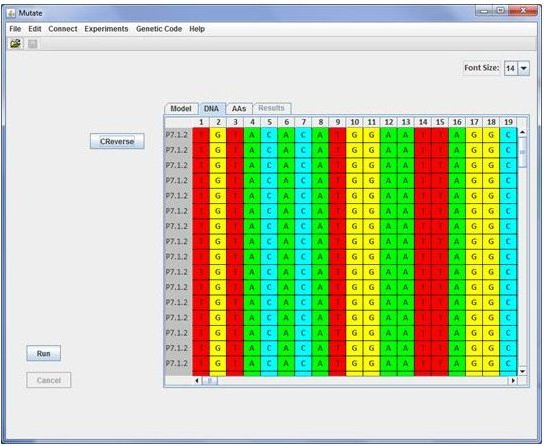MUTATE: IN SILICO PERFORMANCE OF THE FLUCTUATION TESTHints
1. After download Mutate requires the Java 5.0 (or higher) Runtime Environment freely available at http://www.java.com.
2. Whatever the operating system, you should be able to run the program just by double clicking on the mutate.jar file
3. WINDOWS USERS: If double-clicking the .jar file does not open the program, make sure that the jar extension is associated with javaw.exe (in your JRE folder).

Contact
For any questions you can contact me

Mutate Model
The underlying model is similar to the Haldane formulation as described in Zheng (2007) but with differential fitness incorporated for the mutant cells. When implementing the process of bacterial grow it is assumed that if a mutation occurred, the cell divide in one normal and one mutant. This is Kendall's assumption B (Kendall 1960; Zheng 2007). We note b as the mutant fitness or growth rate (Jaeger and Sarkar 1995) then the number of mutants m will increase as

``````
mt+1 = mt *(2*b)

``````

if b = 1 the fitness of mutant and non-mutant is the same. The algorithm for this model is as follows:

``````
Let the initial number of cells N0 = 1.
Generation t: we have Nt cells with mt  of them being "old" mutants.
Generation t+1:
mt mutant cells are not allowed to remute and just divide to produce
2*b*mt.
Nt  - mt  are normal cells and n of them will experience mutation so
Nt  - mt  -n divide normally i.e. 2*(Nt -mt  -n).
The n new mutants just give one mutant plus one normal (assumption B) per cell
so we have n normal + n new mutants.
If we sum up:
Nt+1  = 2*b*mt + 2*(Nt -mt -n) + n + n, which finally gives
Nt+1  = 2*[Nt - mt*(1-b)] and
mt+1 = 2bmt  + n
``````

Given the above algorithm, the population size follows

``````
Nt  = 2tN0 - (1-b)f(M)

``````

where t is the generation number, b is the fitness (growth rate) of the mutant cells and f(M) is a function of the accumulated number of mutations. If b = 1 the population size is

``````
Nt  = 2tN0

``````

which corresponds to the Haldane's formulation as described in Zheng (2007).

Platting efficiency

Plating efficiency refers to the fraction of mutants that finally form observable colonies. It is equivalent to sampling the culture (Gerrish 2008). If we express plating efficiency as a fraction p from the number of mutants before plating

``````
m't+1 = 2bmt + n
then we sample each mutant for platting with probability p to get an expected final number of
mt+1 = pm't+1
``````

A. Carvajal-Rodriguez - Departamento de Bioquímica Genética e Inmunología - Universidad de Vigo. ( Last update: December 2011)